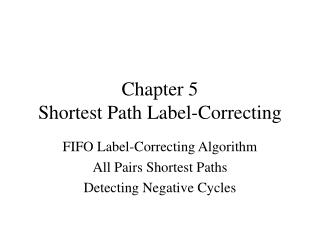DownloadDownload PresentationChapter 5 Shortest Path Label-Correcting

# Chapter 5 Shortest Path Label-Correcting

Télécharger la présentation## Chapter 5 Shortest Path Label-Correcting

- - - - - - - - - - - - - - - - - - - - - - - - - - - E N D - - - - - - - - - - - - - - - - - - - - - - - - - - -
##### Presentation Transcript

1. Chapter 5Shortest Path Label-Correcting FIFO Label-Correcting Algorithm All Pairs Shortest Paths Detecting Negative Cycles

2. Introduction • With negative arc lengths, Dijkstra’s algorithm may fail – why? may need to revise some node labels • Single source goal: • detect a negative cycle if it exists, • otherwise, find shortest paths from s to all other nodes • Label-correcting algorithms maintain a distance label d(j) for every node j • At intermediate nodes, d(j) is an upper bound on the length of a shortest path from s to j • When the algorithm terminates (w/o a negative cycle), d(j) is the shortest path length • No label is permanent until the algorithm stops

3. Optimality Conditions What are necessary and sufficient conditions for a set of labels d(j) to be shortest path lengths? Necessary: (otherwise, we could find a shorter path with i as pred(j) ) These are also sufficient conditions! (proof in text) But if the network contains a negative cycle, then no set of distance labels will satisfy the N&S conditions – for at least some nodes, can always decrease d(j) by going around the negative cycle once more For now, assume no negative cycles; find d(j) that satisfy conditions.

4. FIFO Label-Correcting Algorithm algorithmFIFO label-correcting; begin d(s) := 0 and pred(s) := 0; d(j) :=  for each node j  s; LIST = {s}; while LIST  do begin remove the first element from LIST; for each arc (i, j) A(i) do ifd(j) > d(i) + cijthen begin d(j) := d(i) + cij; pred(j) := i; if j LIST then append j to the end of LIST; end; end; end;

5. Analysis • How do we know the algorithm will stop? • Assume arc lengths are integers. Say C is the largest arc length. Each d(j) is bounded above by nC and from below by –nC. Since each update of d(j) decreases it by at least 1, d(j) will be updated at most 2nC times. Since each iteration updates a distance label, there will be at most 2n2C iterations, or O(n2C) • When it stops, the distance labels will satisfy the optimality conditions • FIFO algorithm actually solves the problem in O(nm) time

6. Detecting a Negative Cycle • Keep track of how many times the algorithm examines each node. • If it examines any node more than n-1 times, then it must be considering paths containing more than n-1 arcs, i.e., paths with cycles. • The only way for a path with more arcs to have shorter total arc length is that it is not a path but a walk containing a negative cycle • Therefore, if algorithm examines a node more than n-1 times, stop and conclude a negative cycle exists

7. Dequeue Version of Label-Correcting • A dequeue is a list to (from) which elements can be added (deleted) at either end • In the dequeue implementation of the label-correcting algorithm, • nodes are selected from the front of the list • nodes are added to the front of the list if they have been examined earlier • nodes are added to the rear of the list otherwise • This implementation is more efficient because it updates distances sooner and reexamines fewer nodes.

8. Detecting Negative Cycles II • Every so often, check to see if the predecessor graph (which is to become the shortest path tree) contains a cycle • such a cycle would occur if a negative cycle were driving the path lengths down • How to detect a cycle in the predecessor graph? • Call the source node labeled and all other nodes unlabeled • Examine each unlabeled node k: • Assign a label k to node k • Trace predecessor indices back from k and assign the label k to all nodes until a previously labeled node, say l, is reached • If nodes k and l have the same label, then the pred graph is cyclic • Requires O(n) time • Do this after every an distance updates

9. All Pairs Shortest Paths • Assume the network is strongly connected and does not contain a negative cycle • Problem: find a shortest path from every node to every other node. For each pair of nodes i and j, we seek d[i, j] = the length of a shortest path from i to j. • For sparse networks, can simply apply the single source shortest path algorithm n times, with a different node as source node each time • For dense networks, an all-pairs label-correcting algorithm is more efficient

10. Floyd-Warshall Algorithm Optimality conditions: A set of node labels {d[i, j]} represent shortest path lengths if and only if they satisfy the “triangle inequality”: Floyd-Warshall updates distances in a clever way until they satisfy the optimality conditions : Let be the length of a shortest path from node i to node j if only nodes 1,…, k-1 are used as intermediate nodes. Compute recursively as